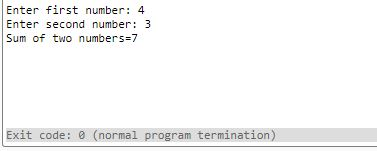UncleCoder.com

UncleCoder.com

Free programming examples and instructions

# CPP Program to Add any two numbers

## CPP Program for how to Add any two numbers

by Krishna

Posted on 27 Jun 2018 Category: c-plus-plus Views: 706

CPP Program to Add any two numbers

This is a simple CPP program to add any two numbers. In this program, the user is asked to enter two  numbers(integers). The result is calculated and displayed on the screen.

``````#include<iostream>
using namespace std;
int main()
{
int num1, num2, sum;
cout<<"Enter first number: ";
cin>>num1;
cout<<"Enter second number: ";
cin>>num2;

// sum of two numbers in stored in variable sum
sum = num1+num2;
// Prints sum
cout<<"Sum of two numbers=" <<sum;
return 0;
}
``````

In the above program the entered two integers are stored in the variable num1 and num2 respectively. These two  numbers are added and sum is stored  in another variable, sum and the value in the variable sum is displayed on the screen.

When the above C++ source code is compile and executed, it will produce the following output:

C code for the same program is also available.

OUTPUT